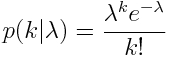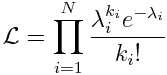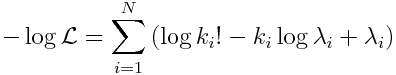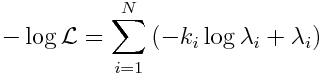# Poisson likelihood

[This is part of a series of modules on optimization methods]

When dealing with count data (for instance, the number of new cases of some disease per unit time, or the number of individuals that enter or leave a population due to death, birth or migration), the Poisson likelihood statistic is often used to assess how well a particular model describes the data.  It is especially appropriate when there are just a few counts per bin, because in that case the Least Squares method is inappropriate.  The Poisson likelihood statistic can in fact be applied to cases where some of the data bins have zero counts.

With the Poisson distribution, the probability of observing k counts in the data, when the value predicted by the model is lambda, isNote that the model prediction, lambda, depends on the model parameters.

If we have a set of N data points, k_i (with i = 1,…,N), the probability (or likelihood) of observing those data points with model predictions for each point, lambda_i , isThe optimal parameters maximize this likelihood.

In practice, it is computationally easier to work with a sum of logs of probabilities rather than their products (to avoid underflow or overflow errors).  In addition, most optimization methods involve minimizing the goodness of fit statistic, rather than maximizing it.  Thus it is standard to deal with the negative log likelihood, which for the Poisson distribution isBecause the log(k_i!) term is independent of the model, it doesn’t affect which model parameters minimize -log(L).  Thus it is usually dropped from the expression for -log(L), to yieldIt is this statistic that is minimized to determine the optimal model parameters that go into the calculation of the lambda_i.  Note that all data can/should be included, even when k_i=0.

Assumptions

The statistic assumes that the data are count data, and the stochasticity about the true model prediction is Poisson distributed.  Most epidemic or population count data are in fact over-dispersed (have more random variation than predicted by the Poisson distribution), in which case a Negative Binomial likelihood statistic is more appropriate.  If in fact the data are over-dispersed and the Poisson negative likelihood statistic is used in parameter optimization, the assessment of the uncertainties on the parameter estimates will be too low.

Visits: 6511